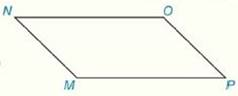Chapter 4.CR, Problem 16CRElementary Geometry For College St...

7th Edition
Alexander + 2 others
ISBN: 9781337614085

Solutions

Chapter
SectionElementary Geometry For College St...

7th Edition
Alexander + 2 others
ISBN: 9781337614085
Textbook Problem

Review Exercises Given: ▱ M N O P m ∠ M = 4 x m ∠ O = 2 x + 50 Find:   m ∠ M   and   m ∠ PTo determine

To Find:

The measures of angles M and P of the parallelogram MNOP with the help of provided information.

Explanation

Properties Used:

1. Two consecutive angles of a parallelogram are supplementary.

2. The opposite angles of a parallelogram are congruent.

Calculation:

It is given that MNOP is a parallelogram with mM=4x and mO=2x+50.

In the parallelogram MNOP, M and O are opposite angles.

By the property ‘opposite angles of a parallelogram are congruent’, mM=mO.

4x=2x+50

2x=50

x=25°

Substituting the value of x in measure of angle M,

mM=4x=4

Still sussing out bartleby?

Check out a sample textbook solution.

See a sample solution

The Solution to Your Study Problems

Bartleby provides explanations to thousands of textbook problems written by our experts, many with advanced degrees!

Get Started

Convert the expressions in Exercises 8596 radical form. (x1/2y1/3)1/5

Finite Mathematics and Applied Calculus (MindTap Course List)

What are the two requirements for a random sample?

Essentials of Statistics for The Behavioral Sciences (MindTap Course List)

In Exercises 4144, determine whether the statement is true or false. 41. 3 20

Applied Calculus for the Managerial, Life, and Social Sciences: A Brief Approach

Evaluate the integral. 27. 15lnRR2dR

Single Variable Calculus: Early Transcendentals

Let f(x)={2x2forx13forx1. Which limit does not exist? a) limx3f(x) b) limx1+f(x) c) limx1f(x) d) limx1f(x)

Study Guide for Stewart's Single Variable Calculus: Early Transcendentals, 8th

True or False: Let . Then f(x, y) is a joint probability function.

Study Guide for Stewart's Multivariable Calculus, 8th